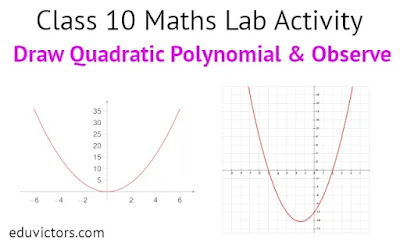## Pages

Showing posts with label class10-maths. Show all posts
Showing posts with label class10-maths. Show all posts

# National Mathematics Day

National Mathematics Day is observed annually on December 22nd to mark the anniversary of the eminent mathematician Srinivasa Ramanujan's birth.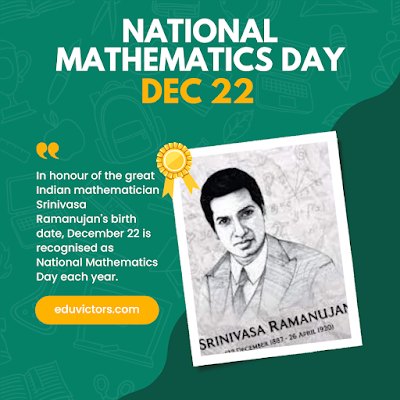# Class 10 Maths MCQs

Q1. A box contains cards numbered 6 to 50. A card is drawn at random from the box. The probability that the drawn card has a number which is a perfect square like 4,9... is

(a) 1/45

(b) 2/15

(c) 4/45

(d) 1/9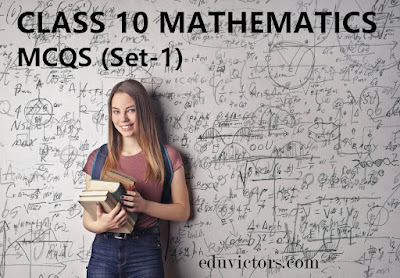# CBSE Class 10 Maths Sample Question Papers 2022-23

Mathematics promotes logical thinking and mental rigour and is a useful method for developing mental discipline. Additionally, comprehending mathematics is essential for learning other academic disciplines like physics, social studies, and even music and art.

CBSE has introduced competency based questions to connect students with real-life applications in mathematics to establish connectivity with 21st Century Skills.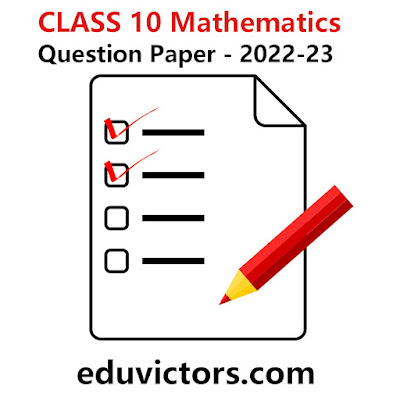# Maths Tip: What are cyclic numbers?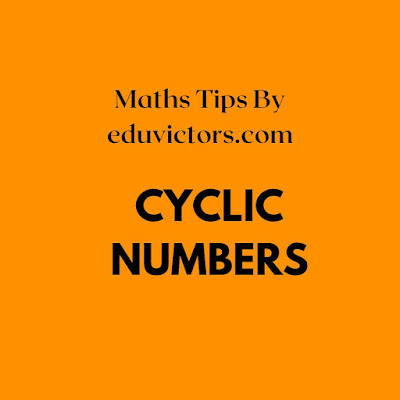# Real Numbers - Quick Tips - Class 10 Maths

Here the video provides quick tips on real numbers that will help you solving very short answer based questions commonly asked in Maths examination.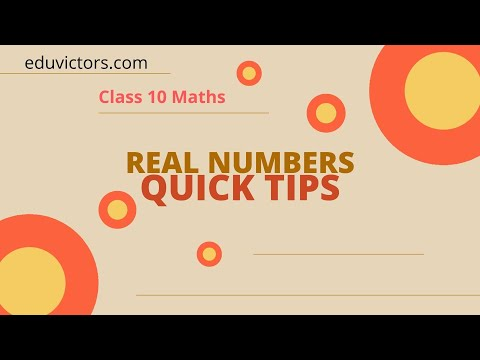# Maths Tip - 1 : Find a rational number and an irrational number between two rational numbers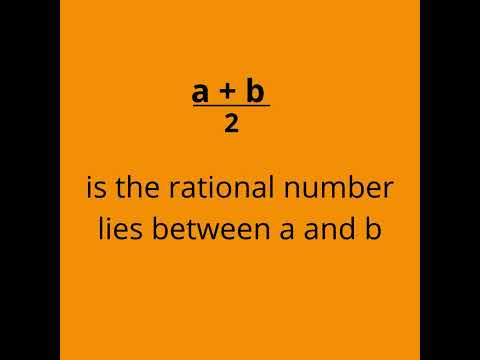# CLASS 10 2022-23 MATHS Detailed Syllabus

CH-1: Real Numbers

Deleted- Euclid’s Division Lemma (Eg- 2,3) & (Exercise 1.1- Q 2,4,5)

CH-2: Polynomials

Deleted- Division Algorithm for Polynomials.

Pg 33 onwards deleted & Exercise- 2.3 deleted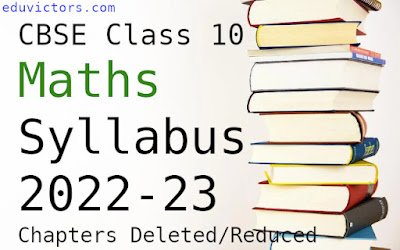# CLASS IX-X: DELETED CHAPTERS IN MATHS AND SCIENCE IN SYLLABUS (2022-23)

Please note the Board will conduct the annual scheme of assessment at the end of the Academic Session 2022-23

Class 9 Science

Deleted Chapters:

- DIVERSITY IN LIVING ORGANISMS

- NATURAL RESOURCES

- WHY DO WE FALL ILL?

Chapter: NATURAL RESOURCES will be taught in the class and  students are encouraged to prepare a brief write up on any concept of this chapter in their Portfolio (internal assessment)# Class 10 Maths - Term 2 Examination Study Notes

Here you will find CBSE Class 10 Maths study materials, MCQs, and Previous Year Question Papers. This study material contains all necessary materials that are not only beneficial for active study of the subject but also crucial for the preparation of the Term 2 Board Exam. You will find Sample Papers, Important Questions, NCERT Solutions, NCERT Exemplar Problems and Solutions, chapter-wise MCQs, Case Study Questions, Exam Preparation Tips, and other relevant articles here, all of which will assist you in better understanding the concepts and becoming more knowledgeable about the subject. All of the materials are completely free.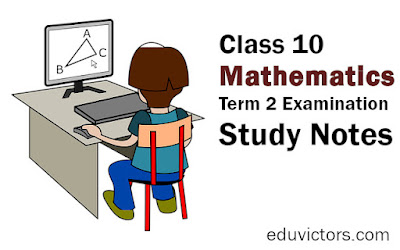# CBSE Class 10 - Applications of Trigonometry - Short Answer Based Questions

Q1: In the figure below which option a, b and c refers to line of sight?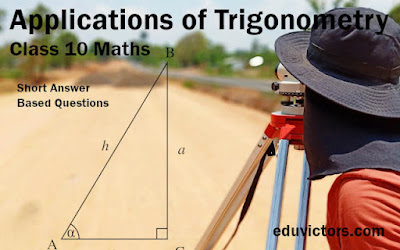# CBSE Class 10 - Mathematics Term 2 (2021-22) Sample Question Papers

Time Allowed: 2 Hours
Maximum Marks: 40

The syllabus covered is:

1. Quadratic Equations + Arithmetic Progressions - 10 Marks
2. Circles  + Constructions - 9 Marks
3. Applications of Trigonometry - 7 Marks
4. Surface Areas and Volumes - 6 Marks
5. Statistics - 8 Marks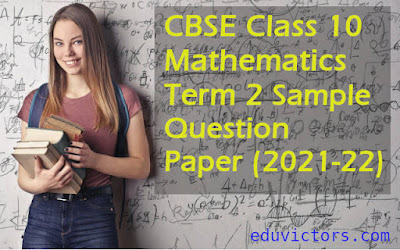# Class 10 Mathematics -  Quadratic Equations Quiz

Choose the correct option from the given choices in each question.

Q1.  The quadratic equation ax2 + bx + c = 0 will have zero number of real zeroes if-

1. b2 > 4ac
2. b2 = 4ac
3. b2 < 4ac
4. none of these
Q2. A quadratic equation ax2 + bx + c = 0 with real roots can have-
1. two linear factors
2. one linear factor
3. three linear factors
4. four linear factors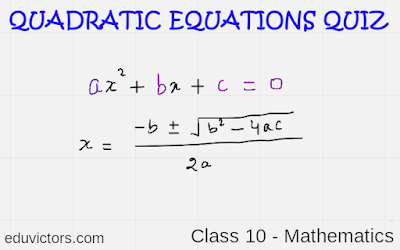# Class 10 Term 2 Sample Question Papers Released By CBSE (2021-22)

CBSE (Central Board of Secondary Education) has released the Sample Question Papers for Class 10 and Class 12 Term 2 in the academic year 2021-22.

CBSE (Central Board of Secondary Education) has released the Sample Paper for Class 10 12 Term 2 Exams 2022. CBSE released the sample papers on their official website for both the Classes 10th and 12th and these papers are in a subjective format.

Students can check the sample paper at  https://cbseacademic.nic.in/

These papers are in a subjective format.# National Mathematics Day

India celebrates National Mathematics Day on December 22 every year. The day marks the birth anniversary of famous mathematician Srinivasa Ramanujan.

*Typo in the graphic new = knew# CBSE Class 10 Maths - Quadratic Equations - Nature of Roots (Solved Problems)

We know that A polynomial p(x) = ax² + bx + c of degree 2 is called a quadratic polynomial, then p(x) = 0 is known as quadratic equation.

There are three methods to find the solution of a quadratic equation:

1. Factorisation method

2. Method of completing the square

What is a Discriminant of Quadratic Equation ax² + bc + c = 0 ?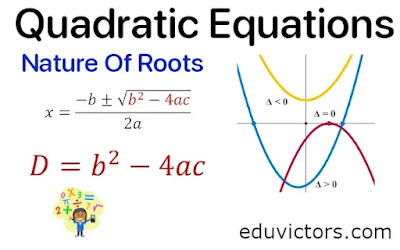# CBSE Class 10 Maths - Area related to Circles (MCQs)

Q1: The circumference of a circle is equal to the perimeter of a square of side 11 cm. Find the area of the circle?

(a) 122 cm²

(b) 144 cm²

(c) 154 cm²

(d) 180 cm²

Q2: The radii of the two circles are 19 cm and 9 cm respectively. Find the radius of a circle that has a circumference equal to the sum of their circumferences.

(a) 28 cm

(b) 10 cm

(c) 44 cm

(d) 22 cm# Class 10 Maths (Basic) Term 1 MCQs For Practice (Set-1)

No. of Questions: 25                                                                             Duration: 30 min

Tough Level: Easy

Q1: The largest number that will divide 398, 436 and 542 leaving remainders 7, 11 and 15 respectively is

(a) 17

(b) 11

(c) 34

(d) 45# Class 10 - Sample Question Papers For Term1 (2021-22) By CBSE

Here follows the PDF download links for Sample Question Papers  and Marking Scheme for Class 10 Subjects for Term 1 Examination (2021-2022).

Duration of Question Paper is 90 minutes and maximum marks is 40

In 90 minutes you need to attempt 40 MCQs.

1. The question paper contains three parts A, B and C.

2. Section A consists of 20 questions of 1 mark each. Attempt any 16 questions.

3. Section B consists of 20 questions of 1 mark each. Attempt any 16 questions.

4. Section C consists of 10 questions based on two Case Studies. Attempt any 8 questions.

5. There is no negative marking.# Class 10 Maths Probability (Assignment)

Complete the following statements with suitable word(s).

1. Probability of an event E + Probability of the event ‘not E’ = _______ .

2. The probability of an event that cannot happen is ________ . Such an event is called ___________ .

3. The probability of an event that is certain to happen is ________ . Such an event is called ___________ .# CBSE Class 10 Maths Lab Activity - Draw Quadratic Polynomial and Observe

OBJECTIVE: To draw the graph of a quadratic polynomial and observe.

(i) The shape of the curve when the coefficient of x² is positive.

(ii) The shape of the curve when the coefficient of x² is negative.

(iii) Its number of zeroes.

MATERIAL REQUIRED

Cardboard, graph paper, ruler, pencil, eraser, pen, adhesive.